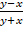Chapter 15.9, Problem 25E

Chapter
Section
Textbook Problem

Evaluate the integral by making an appropriate change of variables.25. ∬ R cos () dA, where R is the trapezoidal region with vertices (1, 0), (2, 0), (0, 2), and (0, 1)

To determine

To evaluate: The integral by change of variable method.

Explanation

Given:

The integral is Rcos(yxy+x)dA where the trapezoidal region with vertices (1,0),(2,0),(0,2) and (0,1).

Property used: Change of Variable

Change of Variable in double integral is given by, Rf(x,y)dA=Sf(x(u,v),y(u,v))|(x,y)(u,v)|dudv (1)

Definition used: Jacobian transformation

(x,y)(u,v)=|xuxvyuyv|=xuyvxvyu

Calculation:

From the given integral observe and let u=yx and v=y+x.

Obtain the value of |(x,y)(u,v)|.

Find the partial derivative of x  and y with respect to u and v. u=yx then ux=1 and uy=1 and v=y+x then vx=1 and vy=1.

T1=(u,v)(x,y)=|uxuyvxvy| (2)

Substitute the corresponding values in equation (2),

T1=|1111|=1(1)1(1)=1+1=2

This is in the form of inverse of Jacobian transformation (T1) but obtain Jacobian transformation (T). Therefore the value of Jacobian 2 will be 12

Substitute the given vertices of trapezoidal in u=yx and v=y+x to get the image of the trapezoidal with vertices that is (1,1),(2,2),(2,2) and (1,1)

Still sussing out bartleby?

Check out a sample textbook solution.

See a sample solution

The Solution to Your Study Problems

Bartleby provides explanations to thousands of textbook problems written by our experts, many with advanced degrees!

Get Started

Find more solutions based on key concepts